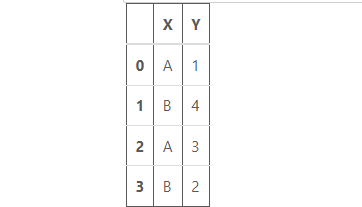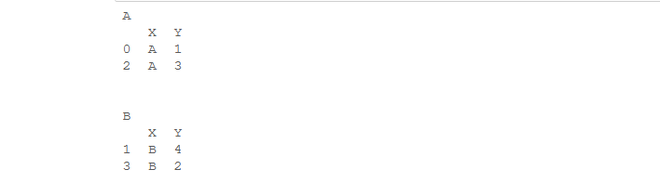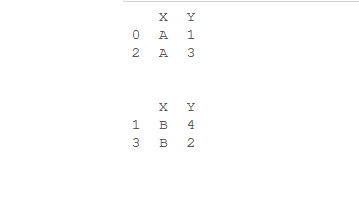# How to Iterate over Dataframe Groups in Python-Pandas?

• Last Updated : 13 Sep, 2022

In this article, we’ll see how we can iterate over the groups in which a data frame is divided. So, let’s see different ways to do this task.

First, Let’s create a data frame:

## Python3

 `# import pandas library``import` `pandas as pd` `# dictionary``dict` `=` `{``'X'``: [``'A'``, ``'B'``, ``'A'``, ``'B'``],``        ``'Y'``: [``1``, ``4``, ``3``, ``2``]}` `# create a dataframe``df ``=` `pd.DataFrame(``dict``)` `# show the dataframe``df`

Output:Iterate over Data frame Groups in Python-Pandas

## Using DataFrame.groupby()  to Iterate over Data frame Groups

DataFrame.groupby() function in Python is used to split the data into groups based on some criteria.

## Python3

 `# import pandas library``import` `pandas as pd` `# dictionary``dict` `=` `{``'X'``: [``'A'``, ``'B'``, ``'A'``, ``'B'``],``        ``'Y'``: [``1``, ``4``, ``3``, ``2``]}` `# create a dataframe``df ``=` `pd.DataFrame(``dict``)` `# group by 'X' column``groups ``=` `df.groupby(``"X"``)` `for` `name, group ``in` `groups:``    ``print``(name)``    ``print``(group)``    ``print``(``"\n"``)`

Output:Iterate over Data frame Groups in Python-Pandas

In above example, we have grouped on the basis of column “X”. As there are two different values under column “X”, so our data frame will be divided into 2 groups. Then our for loop will run 2 times as the number groups are 2. “name” represents the group name and “group” represents the actual grouped data frame.

## Using Dataframe.groupby() and Groupby_object.groups.keys() together

Groupby_object.groups.keys() method will return the keys of the groups.

## Python3

 `# import pandas library``import` `pandas as pd` `# dictionary``dict` `=` `{``'X'``: [``'A'``, ``'B'``, ``'A'``, ``'B'``],``        ``'Y'``: [``1``, ``4``, ``3``, ``2``]}` `# create a dataframe``df ``=` `pd.DataFrame(``dict``)` `# group by "X" column``groups ``=` `df.groupby(``'X'``)` `# extract keys from groups``keys ``=` `groups.groups.keys()` `for` `i ``in` `keys:``    ``print``(groups.get_group(i))``    ``print``(``'\n'``)`

Output:Iterate over Data frame Groups in Python-Pandas

In above example, we’ll use the function groups.get_group() to get all the groups. First we’ll get all the keys of the group and then iterate through that and then calling get_group() method for each key. get_group()  method will return group corresponding to the key.

My Personal Notes arrow_drop_up